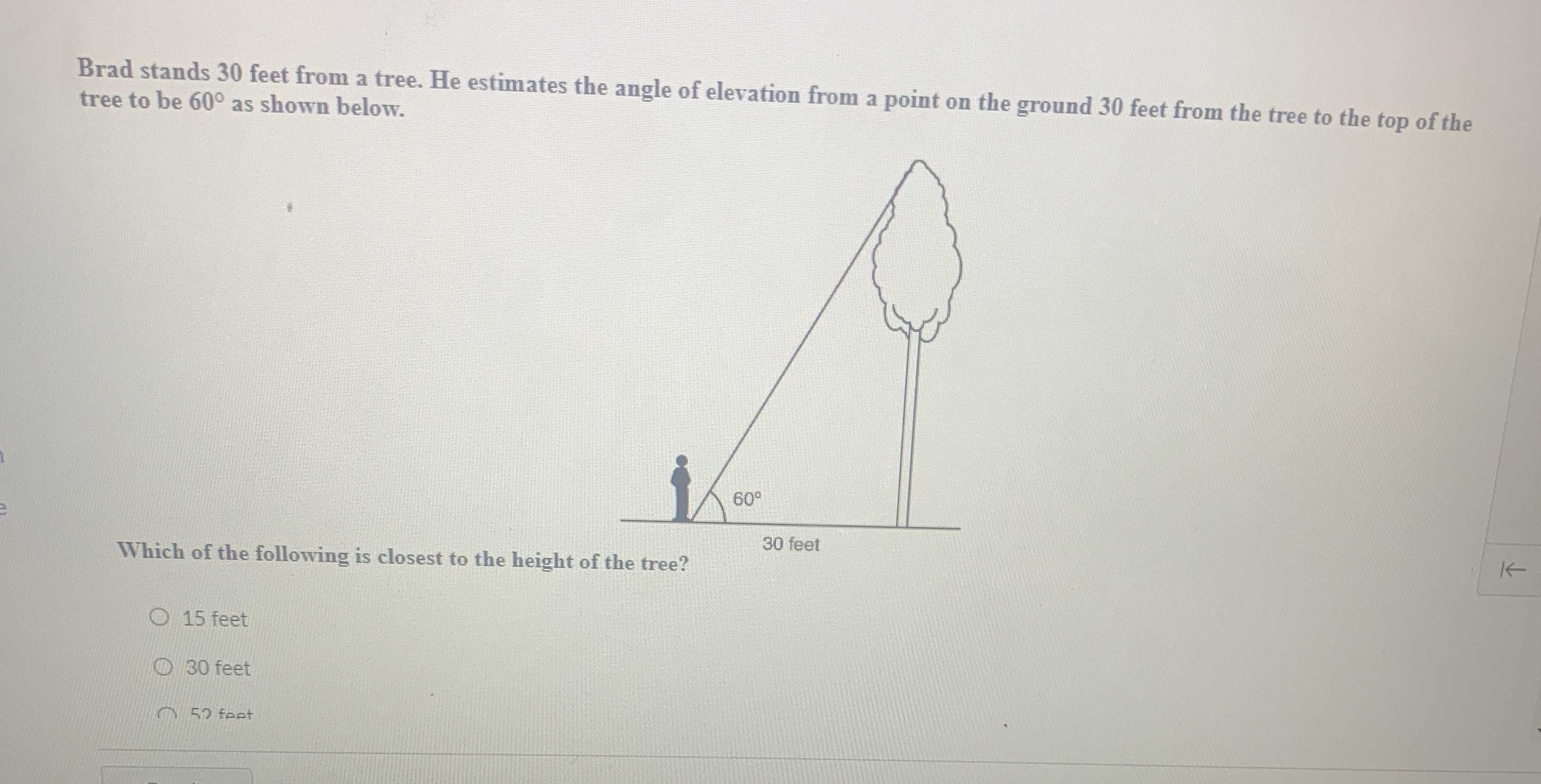### Still have math questions?

Trigonometry
QuestionBrad stands $$30$$ feet from a tree. He estimates the angle of elevation from a point on the ground $$30$$ feet from the tree to the top of the tree to be $$60 ^ { \circ }$$ as shown below.

Which of the following is closest to the height of the tree?

$$tan60 = \frac { opposite} { adjacent} = \frac { treeheight} { distancefromtree} \\ treeheight = tan60\times distancefromtree = 30\sqrt { 3} = 51.9615242 = 52 feet$$